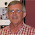## Thursday, June 6, 2013

### Bootstrapping Time Series Data* Every so often someone asks about how to bootstrapping time series data.

* The answer is not either easy or clear (at least as far as I know though there are many people mroe expert on the subject than I).

* The problem is that time series data is by its nature linearly dependent with itself (auto-correlated).

* Let's see how this looks:

clear

set obs 300

gen t = _n
gen x = 0
gen y = 0
gen u = rnormal()*7.5

local N=_N

* You must fill in the simulated data sequentially
qui forv i=2(1)`N' {
replace x = rnormal() + .75*x[`i'-1] if t==`i'
replace y = x*1.5 + .75*y[`i'-1] + u if t==`i'
}

* Let's see what it looks like
* I will first standardize the two.
egen ystd = std(y)
egen xstd = std(x)

two (line ystd t) (line xstd t), name(original, replace) legend(off) ///
title("We can see that the two series are moving together")

* In order to estimate the relationship between y properly we must account for the linear depenent structure of the data.
reg y x

* Is obviously very biased.
gen yl = y[t-1]

* Now we can see that our estimate on x is much closer to our true (1.5).
reg y yl x

* It is worth noting that at this point it is completely unnecccesary to included any lagged values of x.
* Though this is only because we are assuming contemporaneous exogeneity between y and x.

* Now let's make the obvious mistake and try to boostrap.
bs: reg y yl x

* Wow, our estimates look great!  .... Not quite.

* The problem is we are no longer trully bootstrapping our data because lagged values of y are not lagged values of y in our new distributions.

* Drawing on a previous post:
* http://www.econometricsbysimulation.com/2012/07/write-your-own-bootstrap-command.html

cap program drop bootme
program bootme
\$nq preserve
syntax anything [, Reps(integer 100) c1(string) c2(string) c3(string) Keep(string) Noisily]
if "`noisily'" == "" local nq quietly
if "`noisily'" != "" local nq
`nq' gen bootmatcher = _n-1
`nq' gl boot_N=_N
tempfile drawfile
`nq' save `drawfile' , replace
`nq' clear
`nq' set obs `reps'
foreach v in `keep' {
local vtemp=strtoname("v`v'")
`nq' gen `vtemp'=.
}
tempfile returnlist
`nq' save `returnlist' , replace
di as text _newline "Bootstrap replications ({result:`reps'})"
di "----+--- 1 ---+--- 2 ---+--- 3 ---+--- 4 ---+--- 5"
forv i=1/`reps' {
clear
`nq' set obs \$boot_N
`nq' gen bootmatcher = int(runiform()*\$boot_N)
`nq' sort bootmatcher
`nq' merge m:1 bootmatcher using `drawfile', keep(3)
* Making a slight modification to the code her
gen tempvarzzz = rnormal()
sort tempvarzzz
`nq' `c1'
`nq' `c2'
`nq' `c3'
`nq' `c4'
`nq' `c5'
`nq' `c6'
drop tempvarzzz

`nq' use `returnlist', clear
foreach v in `keep' {
local vtemp=strtoname("v`v'")
`nq' replace `vtemp'=`v' if _n==`i'
}
`nq' save `returnlist', replace
if `i'/50 == int(`i'/50) di ".  " `i'
else di _continue "."
}
sum
`nq' restore
gen tempvarzzz = rnormal()
sort tempvarzzz
`c1'
`c2'
`c3'
`c4'
`c5'
`c6'
drop tempvarzzz
end

* We can see that we get basically the same results.
bootme 1, r(200) k(_b[x]) c1(reg y yl x)

* However, now let's add a line of code that generates y lagged as a function of the boostrapped data.
bootme 1, r(200) k(_b[x]) c1(gen ylboot = y[t-1]) c2(reg y ylboot x)

* In this formulation, unsurprisingly ylboot has almost no explanatory power.
gen tboot = _n

two (line ystd tboot) (line xstd tboot), name(boot1, replace) ///
title("Boostrapped data is not autocorrelated")  legend(off)

graph combine boot1 original, rows(2)

* We can see that clearly bootstrapping our results in this way is not going to work.

* One of my classmates is using a method that I think he got from the literature which is a little more complicated.

* First he estimates his model.

reg y yl x
* Then predicts the fitted and residuals

predict yhat
predict uhat, resid

* Then he bootstraps those values and reconstructs the y values from the fitted values by randomly drawing the errors.

* Let's see if we can accomplish this with the previous command.

* First we need to save the fitted values to be grabbed later.
global N=_N
forv i=1(1)\$N {
gl yhat`i' = yhat[`i']
gl x`i' = x[`i']
}

* Write a small program to load yhat values back into the memory
cap program drop datasetup
program datasetup
cap gen yhat0 = .
cap gen ynew = .
cap gen ynewlag = .
cap gen xnew = .

forv i=1(1)\$N {
qui replace yhat0 = \${yhat`i'} if _n==`i'
qui replace xnew = \${x`i'}  if _n==`i'
if `i'>1 qui replace ynew = yhat0+uhat
}
replace ynewlag=ynew[_n-1]
end

datasetup
corr y ynew x xnew
* Initially these values will be identical

bootme 1, r(200) k(_b[x]) c1(datasetup) c2(reg ynew ynewlag xnew)
* As with my classmate's results, the new confidence interval for the x coefficient does not even include the original estimate ~1.2.

* Looking at the data generated in this method
egen ynewstd = std(ynew)
egen xnewstd = std(xnew)

replace t=_n
two (line ynewstd t) (line xnewstd t), name(original, replace) legend(off) ///
title("The new time series")

* Clearly this method is not accomplishing what we would like it to accomplish from a perspective of replicating the estimated coefficients.

* However, interestingly (though not neccessarily believably) the standard errors from the bootstrap are close to the size of the standard errors from the OLS regression above reg y y1 x

* Hope this is helpful.  I will send my friend this link to see if he has anything to add.

#### 1 comment:

1.Francis - check out the block bootstrap and its extensions.